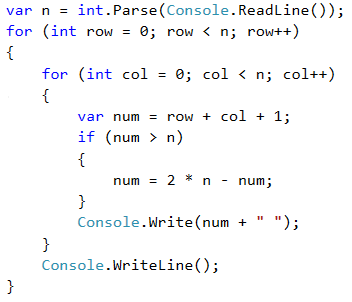# Problem: Numbers Table

Print the numbers 1 … n in a table as in the examples below.

## Sample Input and Output

Input Output Input Output
3 1 2 3
2 3 2
3 2 1
4 1 2 3 4
2 3 4 3
3 4 3 2
4 3 2 1

## Video: Table with Numbers

Watch this video lesson to learn how to print a table of numbers like the shown above using nested loops: https://youtu.be/DVf7riptCwA.

## Hints and Guidelines

We can solve the problem using two nested loops and little calculations inside them:

• We read from the console the table size in an integer variable n.
• We create a for loop that will be responsible for the rows in the table. We name the loop variable row and assign it to an initial value of 0. As a condition, we set row < n. The step is 1.
• In the body of the loop we create a nested for loop that will be responsible for the columns in the table. We name the loop variable col and assign it an initial value of 0. As a condition, we set col < n. The size of the step is 1.
• In the body of the nested loop:
• We create a num variable to which we assign the result of the current row + the current column + 1 (+1 as we start the count from 0).
• We check for num > n. If num is greater than n, we assign a new value to num which is equal to two times n – the current value for num. We do this in order not to exceed n in any of the cells in the table.
• We print the number from the current table cell.
• We print a blank line in the outer loop to move to the next row.

## Implementation of the Idea

Here is a sample implementation of the described idea: